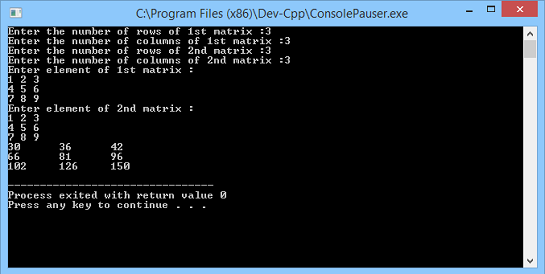# Learning 2-Dimentional Arrays

In this part you will learn: 1. 2D Arrays 2. C syntax 3. Showing output In this tutorial I will teach you about 2D Arrays. We will cover what is a 2D array and how does a 2D array work. What is a 2D Array? In simple words a 2D array is simply and array of array. We have multiple rows and columns in a 2D array. We have learnt previously how we access elements of array by simply accessing the different indexes of array using subscripts. Now similarly we access the different elements of a 2D array by mentioning row number and column number in the subscript to access a specific index. 2D arrays are best used to represent tables as well as matrixes. So today we will see the example of 2D arrays for multiplying two matrices. Multiplication Of Matrix Basic Step: Open Dev C++ then File > new > source file and start writing the code below.
`#include<stdio.h>#include<conio.h>`
These are the header files that we need for coding. The main header is stdio.h which provides us with most of the functions for coding in C and the second header files is just for using a function which can pause the screen until the user hits any key.
`int main(){int matrix1 = { 0 }, matrix2 = { 0 }, matrix_ans = { 0 };	int r1, c1, r2, c2;	int i, j, k;`
For multiplying two matrixes we need three nested for loops which multiply each row of the first matrix to the columns of the second matrix. We will be using three loops because in the rules of matrices we know that if we multiply two matrices e.g 1x3 and 3x2 then the resultant matrix will have 1x2 columns so we will need three loops to traverse through all the rows and columns of all the matrices. To input and output the values of a 2D matrix we need to implement two nested for loops to access each index of a matrix.
`	printf("Enter the number of rows of 1st matrix :");	scanf("%d", &r1);	printf("Enter the number of columns of 1st matrix :");	scanf("%d", &c1);	printf("Enter the number of rows of 2nd matrix :");	scanf("%d", &r2);	printf("Enter the number of columns of 2nd matrix :");	scanf("%d", &c2);`
Now we take input from the user the number of rows and columns of both the matrix.
`if (c1 == r2)	{		printf("Enter element of 1st matrix :\n");		for (i = 0; i < r1; i++)		{			for (j = 0; j < c1; j++)			{				scanf("%d", &matrix1[i][j]);			}		}		printf("Enter element of 2nd matrix :");		for (i = 0; i < r2; i++)		{			for (j = 0; j < c2; j++)			{				scanf("%d", &matrix2[i][j]);			}		}		printf("\nAnswer Matrix is :\n");		for (i = 0; i < r1; i++)		{			for (j = 0; j < c2; j++)			{				matrix_ans[i][j] = 0; 				for (k = 0; k < c1; k++)				{					matrix_ans[i][j] = matrix_ans[i][j] + (matrix1[i][k] *matrix2[k][j]);				}				printf("%d\t", matrix_ans[i][j]);			}			printf("\n");		}	}	}`
Now in this piece of code we have taken input from the user the elements of both the matrix and them calculated their product. First of all we have placed a condition which checks if the number of columns of the first matrix is equal to the number of rows of the second matrix because if this condition is not true then we cannot calculate the product of those two matrixes. After that we have written a nested for loop to take input the elements of array. You see we have placed two subscripts one with a row number and the second with the column number. In this way we access a single index of a 2D array. We have implemented the same kind of nested loop for the second matrix also to take the input. Then comes the nested for loop with three for loops. The first loop controls the row number of the resultant matrix which holds the result of the product. The second loop controls the column number of the resultant matrix as well as the column number of second matrix. The third and the most inner loop controls the column and row number of first and second matrix respectively. This whole nested loop works in a way that is multiplies respective elements of the column of the first matrix to the respective elements of the rows of the second matrix.
`	else	printf("As C1 NOT EQUAL TO R2 SO MULTIPLICATION IS NOT POSSIBLE ");	return 0;}`
The else part executes when the number of column of the first matrix are not equal to the number of rows of the second matrix. Execute > compile then Execute > run Output